The TIMESERIES Procedure

Singular Spectrum Analysis

Subsections:

Given a time series,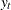, for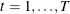, and a window length,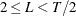, singular spectrum analysis Golyandina, Nekrutkin, and Zhigljavsky (2001) decompose the time series into spectral groupings using the following steps:

Embedding Step

Using the time series, form a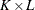trajectory matrix,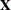, with elements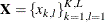such that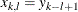for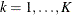and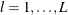and where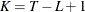. By definition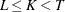, because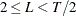.

Decomposition Step

Using the trajectory matrix,, apply singular value decomposition to the trajectory matrix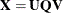where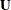represents thematrix that contains the left-hand-side (LHS) eigenvectors, where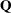represents the diagonal
matrix that contains the singular values, and where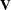represents thematrix that contains the right-hand-side (RHS) eigenvectors.

Therefore,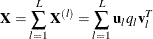where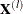represents theprincipal component matrix,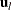represents the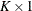left-hand-side (LHS) eigenvector,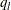represents the singular value, and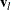represents the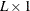right-hand-side (RHS) eigenvector associated with the lth window index.

Grouping Step

For each group index,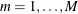, define a group of window indices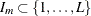. Let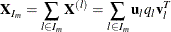represent the grouped trajectory matrix for group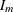. If groupings represent a spectral partition,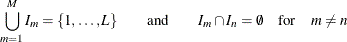then according to the singular value decomposition theory,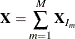Averaging Step

For each group index,, compute the diagonal average of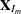,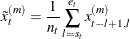where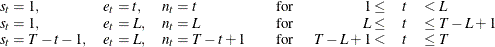If the groupings represent a spectral partition, then by definition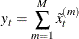Hence, singular spectrum analysis additively decomposes the original time series,, into m component series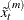for.

Specifying the Window Length

You can explicitly specify the maximum window length,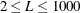, using the LENGTH= option or implicitly specify the window length using the INTERVAL= option in the ID statement or the SEASONALITY= option in the PROC TIMESERIES statement.

Either way the window length is reduced based on the accumulated time series length, T, to enforce the requirement that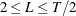.

Specifying the Groups

You can use the GROUPS= option to explicitly specify the composition and number of groups,or use the THRESHOLDPCT= option in the SSA statement to implicitly specify the grouping. The THRESHOLDPCT= option is useful for removing noise or less dominant patterns from the accumulated time series.

Let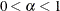be the cumulative percent singular value THRESHOLDPCT=. Then the last group,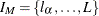, is determined by the smallest value such that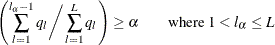Using this rule, the last group,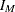, describes the least dominant patterns in the time series and the size of the last group is at least one and is less than the window length,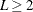.

The magnitudes of the principal components which are plotted using the PLOT=SSA option and selected by the THRESHOLDPCT= option are based on the singular values which appear on the diagonal of. Alternatively, each principal component’s contribution to variation in the series can be quantified by using the squares of the singular values. The relative contributions of the principal components to variation in the series are included in the printed tabular output produced by the PRINT=SSA option.